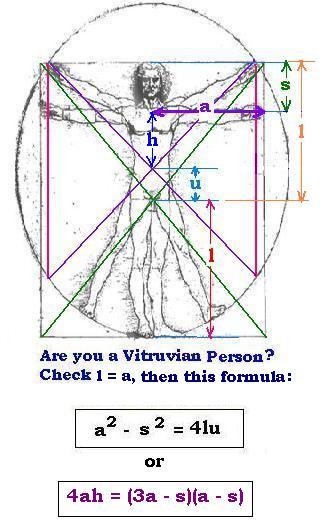Geometry Â  Â  Equations ..

Contents: Â  Definitions | Angles and circles | Triangles

vitruvian man
 Leonardo's vitruvian man Leonardo di ser Piero da Vinci (14521519) had drawn the vitruvian man comming from the architect Vitruvius (Marcus Vitruvius Pollio, around the first century). The body standing is inscribed in a square. With feet and arms outspread, the man is inscribed in a circle.Calculations: Using the square: HD = diagonal/2 HD2 + HD2 = 2 HD2 = (2a)2 Hence: HD2 = 2a2 HD2 = l2 + a2 Then: l2 + a2 = 2a2 Hence: l = a Using the circle: s2 - a2 = r2 - (l - u)2 where r is the radius of the circle. r = l + u, then: a2 - s2 = (l + u)2 - (l - u)2 = (l + u + l - u) x (l + u - l + u) = 2 l x 2 u = 4 ul Hence: a2 - s2 = 4 ul with l = a, we have: a2 - s2 = 4 ua We have also: h = l - u - s ( or a - u - s) u = l - s - h = a - s - h The above formula becomes: (a + s)(a - s) = 4 a (a - s - h) (a + s)(a - s) - 4 a (a - s - h) = 0 (a + s)(a - s) - 4 a (a - s) + 4ah = 0 4 a (a - s) - (a + s)(a - s) = 4ah 4ah = (3a - s)(a - s) 4ah = (3a - s)(a - s)
Â© The Scientific Sentence. 2007.
axiom theorem postulate Lemma corollary